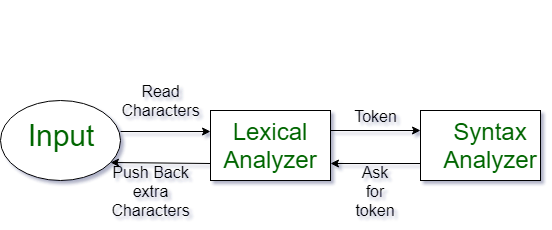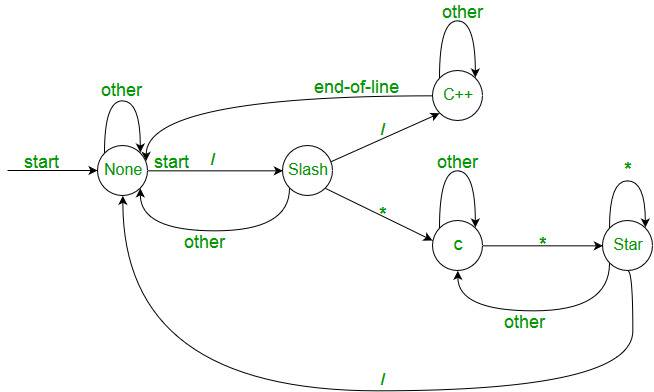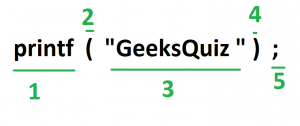Related Articles

# Introduction of Lexical Analysis

• Difficulty Level : Easy
• Last Updated : 28 Jun, 2021

Lexical Analysis is the first phase of the compiler also known as a scanner. It converts the High level input program into a sequence of Tokens.

• Lexical Analysis can be implemented with the Deterministic finite Automata.
• The output is a sequence of tokens that is sent to the parser for syntax analysisWhat is a token?
A lexical token is a sequence of characters that can be treated as a unit in the grammar of the programming languages.

Example of tokens:

• Type token (id, number, real, . . . )
• Punctuation tokens (IF, void, return, . . . )
• Alphabetic tokens (keywords)
```Keywords; Examples-for, while, if etc.
Identifier; Examples-Variable name, function name, etc.
Operators; Examples '+', '++', '-' etc.
Separators; Examples ',' ';' etc```

Example of Non-Tokens:

• Comments, preprocessor directive, macros, blanks, tabs, newline, etc.

Lexeme: The sequence of characters matched by a pattern to form
the corresponding token or a sequence of input characters that comprises a single token is called a lexeme. eg- “float”, “abs_zero_Kelvin”, “=”, “-”, “273”, “;” .

How Lexical Analyzer functions

1. Tokenization i.e. Dividing the program into valid tokens.
2. Remove white space characters.
4. It also provides help in generating error messages by providing row numbers and column numbers.• The lexical analyzer identifies the error with the help of the automation machine and the grammar of the given language on which it is based like C, C++, and gives row number and column number of the error.

Suppose we pass a statement through lexical analyzer –

a = b + c ;                It will generate token sequence like this:

id=id+id;                 Where each id refers to it’s variable in the symbol table referencing all details

For example, consider the program

```int main()
{
// 2 variables
int a, b;
a = 10;
return 0;
}```

All the valid tokens are:

```'int'  'main'  '('  ')'  '{'  'int'  'a' ','  'b'  ';'
'a'  '='  '10'  ';' 'return'  '0'  ';'  '}'```

Above are the valid tokens.
You can observe that we have omitted comments.

As another example, consider below printf statement.There are 5 valid token in this printf statement.
Exercise 1:
Count number of tokens :

```int main()
{
int a = 10, b = 20;
printf("sum is :%d",a+b);
return 0;
}
Answer: Total number of token: 27.```

Exercise 2:

Count number of tokens :

int max(int i);

• Lexical analyzer first read int and finds it to be valid and accepts as token
• max is read by it and found to be a valid function name after reading (
• int  is also a token , then again i as another token and finally ;
``` Answer:  Total number of tokens 7:
int, max, ( ,int, i, ), ;```

Below are previous year GATE question on Lexical analysis.

https://www.geeksforgeeks.org/lexical-analysis-gq/